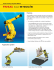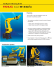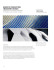# Parametric Robot Control - Association for Robots in Architecture

## Transcription

Parametric Robot Control - Association for Robots in Architecture
```Parametric Robot Control
ABSTRACT
Johannes Braumann
Robots are gaining popularity in architecture. Snøhetta has recently purchased
V i e n n a U n i v e r s i t y o f Te c h n o l o g y
their own industrial robot, becoming one of the first architectural offices to adopt
r o b o t t e c h n o l o g y. A s m o r e a n d m o r e a r c h i t e c t s a r e e x p o s e d t o r o b o t i c f a b r i c a t i o n ,
Sigrid Brell-Cokcan
t h e n e e d f o r e a s y i n t e r o p e r a b i l i t y, i n t e g r a t i o n i n t o a r c h i t e c t u r a l d e s i g n t o o l s a n d
V i e n n a U n i v e r s i t y o f Te c h n o l o g y
general accessibility will increase. Architects are discovering that industrial
robots are much more than kinematic machines for stacking bricks, welding or
milling - they are highly multifunctional and can be used for a huge variety of
t a s k s . H o w e v e r, i n d u s t r y s t a n d a r d s o f t w a r e d o e s n o t p r o v i d e e a s y s o l u t i o n s
for allowing direct robot control right from CAAD (Computer Aided Architectural
Design) systems. In this paper we will discuss existing methods of programming
industrial robots, published architectural results (Gramazio and Kohler 2008) and
the design of a new user interface that allows intuitive control of parametric
designs and customized robotic mass production, by integrating CAM (Computer
Keywords:
robot
programming,
parametric
design,
mass
customization,
grasshopper component design, fabrication, robot milling, digital architecture
242
AC
A
C
CA
DIA
IA 2
20
0
2011
_PROCEEDINGS
INTEGRATION THROUGH COMPUTATION
1
I nt rod uct i o n
T h e r ap i d d evelopment of CA A D softwa re in the la st few d eca d es a llo ws a r chit ect ur a l
f or m s to b e pa ra metrica lly controlled (Fi gure 1) , r esult ing in a ut o ma t ic g ener a t io n
of d e s i gn va ria nts or overa ll design ma na geme nt (E a st ma n et a l. 2 0 0 8 ). R ea lizing
p ar am e t r i c designs requires flexible processes t ha t ca n fa b r ica t e hig h v o lumes a t
r e as on ab l y low costs, referred to a s ma ss cust o mi za t i o n (P iller 2 0 0 4 ; R a ma ni et a l.
2004).
A r c h i te c ts wa nt to control the construction a nd fa b r ica t io n p r o cess o f t heir o wn
d e s i gn s , th e ma chinic processing (Gra ma zio & K o hler, M eng es, S cheur er in K o la r ev ic
2 0 0 8 ; Iw am oto 2009). One problem a rises a s so o n a s (p a r a met r ic) fr eefo r m d esig ns
ar e to b e m ateria lized: a rchitects do not ha ve a p p r o p r ia t e so ft wa r e t o co nt r o l t he v er y
e n d of th e overa ll design process: fa brica tion . L ea d ing CA A D d ev elo p er s ha v e so
f ar n ot p r ov ided a dequa te solutions for directly link ing d iffer ent t y p es o f ma chines t o
C AD s y s t e ms. The building industr y involved in fr eefo r m a r chit ect ur e ha s o v er co me
t h i s d e f i c i t by developing their own “ in-house” cu st o m- ma d e so ft wa r e t o o ls. Ther efo r e
Fig. 1
h i gh -e n d geometr y a nd fa brica tion consulting in a r chit ect ur e is r a p id ly b eco ming a new
s p e c i al i z e d core business run by computer scient ist s a nd ma t hema t icia ns (r efer t o
c om p an i e s such a s Evolute (Eigensa tz et a l. 2010 ) o r d esig n- t o - p r o d uct io n (S cheur er
2 0 1 0 ) ) . In our previous resea rch we ha ve shown th e a d v a nt a g es o f p r o d uct io n- imma nent
d e s i gn an d rea ltime feedba ck to eva lua te not on ly t he d esig n o f a p a r a met r ic o b ject ,
b u t al s o i ts ma nufa cturing rela ted properties (Bre ll- Co k ca n a nd B r a uma nn 2 0 1 0 ).
In t h i s p ap er we propose the use of a gra phical a lg o r it hm ed it o r a s a new int er fa ce
Fig. 2
f or an i n d u stria l robot. The result is user-custom ized CA A D so ft wa r e wher e ma chine
c on s tr ai n t s a re included a s a dditiona l design pa r a met er s d ir ect ly g ener a t ing r o b o t
c on t r ol d ata files, to be used for ma ss customiza t io n.
2
E xi s t i ng Met hod s fo r Pro gram m i ng a KU KA R ob ot
In ge n e r al , there a re two distinctive stra tegies for p r o g r a mming ind ust r ia l r o b o t s: O nline/
Fig. 3
au tom at i c (Fi gure 2) a nd offline/ma nua l progra mming ( Fi gu re 3 ) (B ig g s a nd M a cDo na ld
2 0 0 3 ) . O n l i ne progra mming is used mostly for ind ust r ia l a p p lica t io ns such a s lo a d ing
an d w e l d i n g , where points a re “ ta ught” individually v ia t he K CP (K U K A Co nt r o l P a nel)
or i n t h e v i r tua l robot environment KUKA SimPro. In t he field o f a r chit ect ur e, wher e t he
d i gi tal C AD/CA M workflow is importa nt, online pr o g r a mming is r a r ely used .
2.1
ROBOT OFFLINE PROGRAMMING
Rob ot of f l i n e progra mming is performed a t a n exte r na l co mp ut er o ut sid e t he r o b o t ic cell
an d i s s i m i l a r to the tra ditiona l wa y of working wit h CNC (Co mp ut er Numer ic Co nt r o l)
m ac h i n e s , (i.e., moving da ta from CA D to CA M so ft wa r e) a nd t hen a s NC (Numer ic
C on t r ol ) c ode, a lso known a s G-Code, to a CNC milling ma chine. A d d it io na lly, r o b o t
m i l l i n g r e q uires the use of a kinema tic post-pro cesso r /simula t o r, in o ur ca se K U K A
Si m P r o/ C A MRob. This results in a digita l workflo w inv o lv ing a t lea st t hr ee so ft wa r e
t ool s b y d i f ferent developers. “ Rea ltime” design a nd p r o d uct io n feed b a ck t ha t a llo ws
an e v al u ati o n of the fa brica tion properties throug ho ut t he wo r k flo w is missing .
P r e v i ou s r e sea rch shows the possibility of strea mlining t he d ig it a l CA D- CA M - R o b o t
w or k f l ow b y using pa ra metric design tools for robo t milling (B r ell- Co k ca n et a l. 2 0 0 9 ).
To f u r t h e r optimize offline progra mming for a K U K A r o b o t we will d emo nst r a t e in
Se c t i on 4 t he design of the custom KUKA | prc (p a r a met r ic r o b o t co nt r o l) co mp o nent
w i th i n G r as shopper, a pa ra metric design plug-in fo r t he CA D so ft wa r e R hino cer o s.
2.2
KU K A R O B O T L A N G U A G E C O D E
Figure 1. Spencer Dock Bridge: Parametric
The KUKA Robot Language (KRL) is a proprietary programming language with similarities to
freeform design, CNC-milled formwork - Image
courtesy of Nedcam
Pascal and is used to control KUKA robots. Unlike G-Code, it does not contain just tool and
Figure 2. Online programming via KUKA Sim Pro
machine movement commands, but can also declare variables and work with conditional
(left) or via the KUKA Control Panel (right)
clauses. While the KRL documentation (KUKA Robotics 2003) explains a set of commands, it
Figure 3. Existing offline data workflow from CAD to
an industrial robot
does not provide detailed information on the required structure of a proper control data file in
243
INTERFACE AND IMMERSION
Fig. 4
KRL (Mühe et al. 2010). We therefore had to reverse-engineer the structure of KRL files created
by the kinematics postprocessor KUKA SimPro/CAMRob. Basically, a Numeric Control file for
KUKA consists of two separate files: A *.dat file where variables such as the home position
are stored and a *.src file which contains the various commands, like movement commands,
loops, sensor queries et cetera. There are various ways of giving movement commands to a
robot, the most common ones are the following (Takase et al. 1981):
Joint coordinate programming consists of absolute axis rotation commands, instructing the
robot to move each of its six axes to a defined rotation value.
A1 0, A2 10, A3 90, A4 20, A5 60, A6 25
Cartesian coordinate programming defines the location and orientation of the end-effector
in a previously defined Cartesian coordinate system. This can either be point-to-point (PTP)
commands where the end-effector moves from one position to the next with the least amount
of axis rotation or linear (LIN) commands where the end-effector moves from one position to
the next along a straight line.
X 10, Y 20, Z 40, A 45, B 75, C 15
3
Par a me t ri c R ob ot P rogra mmi n g for A rc h i t e c t u ra l De s i gn
Understanding the robot’s data processing described in Section 2, it appears feasible to
create KRL code via custom scripting (offline programming), thereby automating the fabrication
process for architectural mass customization.
For most architectural purposes, the location of the tool has to be precisely controlled,
therefore requir ing t he use o f Ca r t es i a n co o r d i na t e p r o g r a mmi ng . The k inema t ics o f t he
robot do not necessa r ily ha v e t o b e simula t ed in t he p a r a met r ic mo d eling env ir o nment ,
a s they a re so lv ed a cco r d ing t o t he end - effect o r p o sit io n/o r ient a t io n in r ea lt ime when
the KRL code is ex ecut ed a t t he co nt r o l unit . H o wev er, simula t ing t he r o b o t ’s k inema t ics
in a design env ir o nment ena b les t he d esig ner t o r ea ct t o r ea cha b ilit y - co nst r a int s o r
possible collis io ns, t her eb y o p t imizing t he use o f t he a v a ila b le wo r k sp a ce.
3.1
O F F LI N E P R O G R A M M I N G V I A C U S T O M I Z E D S C R I P T I N G
A recent a rch it ect ur a l ex a mp le inv o lv ing ind ust r ia l r o b o t s a nd cust o m scr ip t ing is t he
P ike L oop pro ject ( Fi gu re 4 ) b y G r a ma zio a nd K o hler (B ä r t schi et a l. 2 0 1 0 ), wher e a
specia l “ wigg led b o nd b r ick a r r a ng ement is ma p p ed o nt o a g ener ic d o ub le cur v ed
surfa ce” . This r esult s in a CA D- cr ea t ed sur fa ce b eing p r o cessed b y a scr ip t t ha t
dea ls with the v a r io us p r o b lems inv o lv ed in r o b o t ic b r ick st a ck ing , lik e t he g ener a l
a rra ngement, t he b o und a r y co nd it io ns a nd o p t imiza t io n issues ( Fi gu re 5 a ) . While t he
script is exec ut ed , t he user ca nno t int er a ct b ut ha s t o wa it unt il t he scr ip t is finished ;
the user is then p r esent ed wit h t he r esult a nd ha s t o ev a lua t e if t he a chiev ed o ut p ut
meets predefined r eq uir ement s o r if t he r esult ha s t o b e cha ng ed . This ca n b e d o ne
either by cha n g ing t he p r ed efined r eq uir ement s, b y mo d ify ing t he init ia l CA D g eo met r y,
Figure 4. Robotic fabrication for the Pike Loop
Project by Gramazio and Kohler
or by cha ngin g t he co d e it self. B a sica lly, t he co d e a ct s a s a s o l ver fo r a seq uence o f
clea rly (pre)defined p r o b lems.
244
_PROCEEDINGS
INTEGRATION THROUGH COMPUTATION
Ev e n th ou gh the use of a n industria l robot for brickla y ing is a v er y inno v a t iv e a r chit ect ur a l
ap p l i c ati on , the published digita l workflow via cust o mized scr ip t ing d escr ib es a
ty p i c al ar c hitectura l design process with a des ig ner (t his ca n b e a n a r chit ect ) t o
c r e ate an aesthetic surfa ce in CA D . A progra mm er (sup p o sed ly a co mp ut er scient ist
or m ath e m a ticia n) then solves the “ brickla ying p r o b lem” b y a p p ly ing t he g eo met r ic
c on s t r ai n ts of bricks to a predefined surfa ce (simila r t o t he k no wn seg ment a t io n
p r ob l e m of a ny freeform surfa ce in a rchitecture), (E ig ensa t z et a l. 2 0 1 0 ) fo llo wed b y a
te c h n i c i an who postprocesses the geometric da ta o ut p ut fo r t he r o b o t co nt r o l d a t a file
( e . g. i n a r o bot simula tion progra m), simila r to a co nt r a ct o r who ha s t o p o st p r o cess
r e c e i v e d ge o metric da ta for his CNC-ma chines. The q uest io n a r ises her e ho w t o fur t her
c u s t om i z e the digita l workflow to a llow the user, i. e. , t he d esig ner, t o ma nip ula t e t he
i n i ti al C AD surfa ce, the brick-la yout a nd the robo t co nt r o l simult a neo usly ?
3.2
A NEW APPROACH FOR ROBOT OFFLINE PROGRAMMING
T h e P i k e Loop project shows how customized sc r ip t ing a llo ws a r chit ect s t o a lt er t heir
d e s i gn s p ar a metrica lly in a linea r process a nd ho w t o ex ecut e p a r a met r ic d esig ns wit h
an i n d u s tr i a l robot set-up, in this ca se a robotic g r ip p er. While t he ex emp la r y d a t a
f l ow d e s c r i bed in Section 3.1 is suita ble for ma ny a r chit ect ur a l a p p lica t io ns, we a r g ue
Fig. 5a
th at i t i s n ot idea l for a fluent bottom-up a rchitect ur a l d es i g n t o r o b o t mi l l i ng wo r k flo w.
In ad d i t i on t o the desire of a fully pa ra metric robo t co nt r o l, t he funct io na lit y o f co mmo n
C AM s of tw a re such a s user-defined tool geomet r ies (e. g . v a r y ing t o o l d ia met er o r
c u t t i n g l e n gths) within a single project, a utoma t ed t o o lp a t h g ener a t io n a nd co llisio n
c h e c k i n g h a s to be included in a pa ra metric mod el fo r r o b o t milling .
A w e l l -i n t e g ra ted pa ra metric design tool for robot milling a nd a r chit ect ur a l d esig n (e. g .
f or m as s c ustomiza tion) requires the user (i.e., t he d esig ner ) t o b e a b le t o inst a nt ly
an d c on s tantly intera ct with a ll pa rts of the cod e. P r ep a r ing d a t a fo r r o b o t milling is
t h e r e f or e a highly non-linea r process.
In ou r p r e v i ous resea rch we ha ve shown tha t a fu lly p a r a met r ic d esig n fo r r o b o t milling
gr e at l y s t r e a mlines the ma nufa cturing process a s cha ng es imp o sed b y t o o l g eo met r y,
Fig. 5b
m ate r i al p r operties or ma chine constra ints a re easy t o imp lement . We ha v e p r o p o sed
s e v e r al s t r a tegies to improve the workflow from d ig it a l d esig n t o p r o d uct io n, such a s
i n c l u d i n g m a chine constra ints in the pa ra metric mo d el o r g ener a t ing G - co d e wit h a
G r as s h op p er definition. However, some workflow p r o b lems r ema ined a s t he G - co d e
s ti l l h ad t o be post-processed before sending it t o t he r o b o t ’s co nt r o l unit . R ecent ly,
w e d e m on s tra ted the use of a Gra sshopper definit io n, ca p a b le o f g ener a t ing a ct ua l
KRL c od e . However, ma intena nce of this complex clust er o f G r a ssho p p er co mp o nent s
p r ov e d t o be cumbersome, a s there wa s no po ssib ilit y o f imp lement ing a cent r a l
gr ap h i c al i nterfa ce or intuitive design for ma ss cust o miza t io n. S o lv ing t hese issues
r e q u i r e s th e development of a n a ctua l robot milling p lug - in, a s d emo nst r a t ed b y t he
KU KA |p r c c o mponent in Section 4.1.
4
Des i g ni ng a Param et ri c Co m p o nent f or R ob ot M i l l i n g
T h e m ai n i dea to fa cilita te working with robots is a b o t t o m- up d es i g n wher e r o b o t
m i l l i n g s tr ategies determine the geometric desig n r ig ht fr o m t he b eg inning a nd
t h e r e f or e b ecome a n integra l pa rt of the design, a lwa y s a v a ila b le in t he b a ck g r o und
f or an al y s i s a nd simula tion. I t is possible to robot ica lly fa b r ica t e infinit e d esig n v a r ia nt s
of th e c u r r ent model a t ever y time with just a few mo useclick s a nd wit ho ut ha v ing t o
go t h r ou gh multiple export/import steps from C A D t o CA M t o t he r o b o t ( Fi gu re 3 ).
C ol l i s i on s and insufficient tool length a re detected a t r unt ime, so t ha t t he d esig n (i. e. ,
th e c od e ) c an be insta ntly revised.
G r as s h op p er wa s chosen a s the pa ra metric env ir o nment b eca use o f it s mo d ula r,
op e n s tr u c t ure a nd rea ltime preview. Especia lly imp o r t a nt fo r r o b o t milling is t ha t
Figure 5a. Dataflow comparison - linear versus
G r as s h op p er ’s component system a llows comp a r t ment a lizing so lut io ns a nd r eusing
non-linear processes: conventional scripting
e x i s ti n g c ode, in order to a da pt to emerging desig n r eq uir ement s o r p r o b lems. We
r e f e r t o th e se functiona l groups of components a s mo d ul es ( Fi gu re 5 b ) .
Figure 5a. Integrated parametric approach for
robot milling
245
INTERFACE AND IMMERSION
For exa mple, t he d esig ner ca n cr ea t e mo r e t ha n o nly o ne g eo met r ic mo d ule a nd
immedia tely g ener a t e t o o lp a t hs, simult a neo usly ca lcula t ing t he end - effect o r o r ient a t io n,
checking for co llisio ns a nd cr ea t ing t he K R L co d e fo r ea ch o f t hem, just b y seq uent ia lly
plugging them int o t he t o o lp a t h cr ea t io n mo d ule.
“Conventional” scripting can mostly be assumed to be solving clearly defined problems in
a predefined sequence which also applies to most Grasshopper modules. In co nt r a st t o
typica l scripti ng co d e, ho wev er, t he K U K A | p r c co mp o nent is d efined a s fo llo ws: b y
integra ting robo t co nst r a int s a nd imp o r t a nt CA M funct io ns such a s t o o lp a t h g ener a t io n
into the pa ra met r ic mo d el, we g ener a t e a d esig n t o o l fo r r o b o t milling t ha t inst a nt ly
rea cts to a ll cha ng es, a llo ws fluid a nd int uit iv e mo d ifica t io ns in a ny o f t he mo d ules,
a t a ny time. T his o ffer s t he p o ssib ilit y o f q uick ly ev a lua t ing d esig ns fo r r o b o t milling ,
simula ting too lp a t hs a nd g ener a t ing t he ma chining co d e wit ho ut ha v ing t o d ea l wit h
either hundreds o f lines o f co d e o r sev er a l d iffer ent CA D, CA M a nd k inema t ic so ft wa r e
pa cka ges or p o st p r o cessing . The K R L co mp o nent t her efo r e est a b lishes a feed b a ck
loop from CA AD t o CA M t o t he r o b o t a nd b a ck a g a in.
4.1
K U K A K R L - C O M P O N E N T: G E N E R A L A P P R O A C H
While designing t he K U K A R o b o t L a ng ua g e co mp o nent fo r G r a ssho p p er we a imed t o
fulfill the follo wing fiv e r eq uir ement s:
t
The com p o nent ha s t o int eg r a t e int o t he G r a ssho p p er env ir o nment lik e a
na tive component .
t
I t should fo cus o n flex ib le, d y na mic r o b o t co nt r o l wit ho ut t he fea t ur eoverloa d o f co mmer cia l CA M so ft wa r e.
Fig. 6
t
I ts ca lcula t io ns a r e t o b e co mp let ed in less t ha n a seco nd t o r et a in t he
nea r-realt ime feed b a ck , wit h a n a d d it io na l q uick p r ev iew mo d e.
t
I ts functio na lit y sho uld b e t r a nsp a r ent t o t he d esig ner a nd cust o miza b le,
wra pped in a n a ccessib le user int er fa ce.
t
The output ha s t o co nsist o f huma n- r ea d a b le, no n- b ina r y K R L co d e t ha t
can be executed directly at the robot, without any additional steps such as
post-pro cessing .
The resulting KUKA Robot Language Component for Grasshopper is written using
the .NET framework (RhinoCommon SDK & Grasshopper SDK) in Visual Studio and
seamlessly integrates with the default Grasshopper components. A project in Visual
Studio can contain several classes (i.e., Grasshopper components) which compile
into a single class library that can be easily distributed among users.
4.2
K U K A K R L C O M P O N E N T: F U N C T I O N A L I T Y
We d e f i n e t h e e n d - e f f e c t o r o r i e n t a t i o n a n d p o s i t i o n a l o n g a t o o l p a t h i n t h e K R L
c o m p o n e n t w i t h t h e h e l p o f t h r e e i n p u t s ( F i g u re 6 ) :
t
The tooltipcurve defines the position of the tool center point (TCP) on the
t o o l p a t h d u r i n g a m i l l i n g j o b a t a p o i n t p n.
t
T h e g u i d e c u r v e a t p n’ d e f i n e s t h e t o o l a x i s a s t h e v e c t o r f r o m p n’ t o p n.
t
The orientationpoint q defines the rotation of the end-effector around the
toolaxis and can be either a static point or a dynamic point along a curve.
I n F i g u re 6 , q i s p l a c e d a l o n g t h e r o b o t ’s a x i s A 1 .
Synchronizing point p n ’ and p n is done via the curves’ parameterization or by dividing the
curves into an equal number of points. The resulting information consists of a point (XYZ)
and Euler angles (ABC) which can be formatted and exported as KRL code.
Figure 6. Necessary geometric input for KRL code
generation: tool and guidecurve (left), orientation of
the end-effector (right)
Besides the parametric numeric (simulation slider) and geometrical input (toolpath
points, collision geometry), the KRL component also provides a graphical user
246
_PROCEEDINGS
INTEGRATION THROUGH COMPUTATION
Fig. 7
interface, accessible within Grasshopper (Figure 7). Simulated objects such as the
r o b o t ’s e n d - e f f e c t o r a r e g e n e r a t e d p a r a m e t r i c a l l y w i t h i n t h e c o m p o n e n t a c c o r d i n g
to the tool parameters and do not require separate Grasshopper geometry input.
One of the main problems when using existing CAM software is that CAM software
is a closed system that contains many functions from mechanical engineering,
which are not necessarily required for architectural designs. The options available
within the KRL component focus on the most essential features of CAM software:
t
t
Se t u p the robot’s ba se including the refere nced co o r d ina t e sy st em
t
De f i n e the lea d in a nd lea d out (none/offset /t a ng ent ia l/a lo ng t o o l a x is)
t
Se t t oo l number a nd properties (tool length/ cut t ing leng t h/d ia met er /feed r a t e)
t
C ol l i s i o n control settings
t
V i s u al i za tion/viewport performa nce settings
t
KRL f i l e settings
t
En ab l e/disa ble sequentia l file na ming for ma ss cust o miza t io n
W h e n e v e r t he pa ra metric model is cha nged, the K R L files a r e simult a neo usly up d a t ed
w i t h t h e n ewest version in the director y define d b y t he o ut p ut set t ing s. F o r ma ss
c u s t om i z at i on it is possible to override this set t ing a nd a t t a ch a seq uence numb er
to t h e f i l e n a me so tha t sepa ra te files a re a utoma t ica lly g ener a t ed fo r ea ch v a r ia t io n.
4.3
KU K A K R L C O M P O N E N T: A P P L I E D D E S I G N S A N D E VA L U AT I O N
T h e KU KA K RL component’s ma ss customiza tion ca p a b ilit ies wer e t est ed wit h d iffer ent
p ar am e t r i c designs. One design ta sk wa s to creat e a p a r a met r ic E P S b r ick wa ll o ut o f
i n d i v i d u al , s ta cka ble elements with va r ying openin g s. It s b a sic t o o lp a t hs co nsist o f just
t w o p ar am e tric cur ves tha t crea te a ra nge of differ ent p hy sica l, st a ck a b le 3 D mo d ules
w i th v ar y i n g geometr y a nd openings (Fi gure 8) .
T h e G r a s s h o p p e r d e f i n i t i o n i n F i g u re 8 a l l o w s v a r i o u s r e a l t i m e p r e v i e w s w h i c h
enable the designer to simultaneously follow the design and production process. The
geometric generation of the parametric tooltip- and guidecurves (1), the individual
modules to be produced (2), the stacked results (3), the automated toolpath
generation (4) and a simulated end-effector are all shown at the same time in a single
viewport. The end-effector simulation and KRL output (5) are handled by the new
custom KRL component. This demonstrates our initial idea of using the parametric
Figure 7. KUKA|prc - graphical interface for
design environment as a custom design tool where changes to single geometric
parametric robot control
247
INTERFACE AND IMMERSION
Fig. 8
Fig. 9
modules, or to the overall design, have immediate consequences for the toolpath
generation and vice versa.
In contrast to traditional CAM software, this modular approach allows an open and flexible
parametric CAM environment, where the user does not necessarily have to rely on preset toolpath-strategies, but can substitute these components with his own parametric
Grasshopper definitions.
To compare the Grasshopper integrated KUKA plugin with traditional CAD/CAM, two prototypes
of the same parametric wall design were milled. The physical models of both results were
indistinguishable, while the preparation with the commercial CAM software took only slightly
longer compared to the KRL component. However, any successive variation would widen the
time-gap as the CAD/CAM software has to be set up each time, while the KRL component
can continue generating KRL code instantly. For the design variant in Figure 8, the toolpath
consists of 1500 points. The KRL file is generated within 45ms on a 2GHz laptop, which would
theoretically allow the automated output of 20 design variants per second. This rapid response
allows designers to quickly output and fabricate design variants, without having to manually
process each file in the CAM software and postprocess for the robot´s kinematics. A further
advantage is that the calculations happen parallel to the design process: while the designer can
“play” with the geometric entities such as curves and stackable 3D modules, the KUKA plugin
Figure 8. Production immanent parametric model
provides feedback on fabrication feasibility and possible collisions.
simultaneously showing realtime preview of
geometry input (1), individual modules (2), final
design (3), milling simulation with feasibility analysis
(4) and KRL code (5)
In order to evaluate the Grasshopper-integrated KUKA plugin with a full scale architectural
Figure 9. 12m² freeform surface segmented in 18
panels (left), comparing flank milling and point milling
according to material efficiency (middle, right)
prototype, we revisited “Plug & Play,” a robotically fabricated project from 2009/2010 that
required the fabrication of 18 unique freeform panels for a 12m² freeform interior surface as part
of a children´s playground design (Figure 9). Different milling strategies such as roughening,
finishing and flank milling were evaluated and successfully used with the KUKA plugin.
248
_PROCEEDINGS
INTEGRATION THROUGH COMPUTATION
Fig. 10
Fig. 11
Roughening and finishing are common point milling strategies that use the tooltip of the endmill
to coarsely remove stock material layer by layer (roughening), and then to create the final
surface finish (finishing). In contrast, flank milling (Schindler 2009) utilizes the whole cutting
length of the endmill to tangentially cut finished surfaces out of the stockmodel.
Du e to th e se inherent differences between po int a nd fla nk milling st r a t eg ies, t wo
s e p ar ate approa ches were developed to produce t he sha p e o f t he p a nels. F o r
r ou gh e n i n g/finishing, severa l blocks of XPS were used t o mill t he fina l sur fa ce. F o r
f l an k m i l l i n g, the freeform sha pe wa s a pproxima t ed wit h r uled sur fa ces wit h t he wid t h
of e ac h s u r fa ce corresponding to the height of a co mmo n X P S p a nel. A sing le cut wa s
t h e n p e r f or med to produce the finished surfa ces.
Both from the computational and physical point of view, flank milling proved to be the
best solution for this particular project. Compared to the point milling strategies, flank
milling required approximately 80% less toolpath length, which equals machine time, and
achieved more than 40% in material savings (Figure 9). Similar results for flank milling
applications were reported by Waldt (2005).
Figure 10 shows the comparison of the proposed milling strategies of an exemplary panel in
commercial CAM software and the impact of flank milling on toolpath lengths and machine time.
The increased toolpath length of point milling also has consequences for the parametric design
environment, as the robot code generation is noticeably slowed down by the excessive amount
of data. In the freeform playground design only flank milling allows the designer to work in
a fluent, near realtime design environment. The right choice of milling strategies is therefore
crucial for the parametric design environment and efficiency of mass customization. Therefore
the designer has to evaluate milling strategies before creating a parametric model. Any designto-fabrication automation requires milling experience to allow such decisions.
The two realized examples, the parametric wall and the children´s playground design presented
in this paper show that parametric design software with integrated CAD/CAM features is
capable of accommodating customized and optimized fabrication strategies that would not be
possible using traditional CAM software tools only. Significant savings in machining time and
material costs, along with the ecological aspect of producing less waste, can be achieved by
optimizing designs for fabrication instead of just sending the final 3D CAD file to the fabrication
workshop. The KUKA component for Grasshopper enables a fluid workflow from optimized
design to robotic fabrication.
Figure 10. Freeform playground: comparing pointand flank milling strategies
Figure 11. Modular, parametric robot control using
KUKA|prc
249
INTERFACE AND IMMERSION
Fig. 12
Fig. 13
5
Con c l u s i on a n d Ou t l ook
Industrial robots have been applied in full-scale construction – as a replacement for manual labor – in
Japan since the 1980s with little success due to automation difficulties and economic reasons. As
Bechthold (2010) states, the development of automated programming strategies is fundamental for
using robots in “customized construction”.
Our intent in research is to enable a wide range of architects in the use of robots with a similar ease of
use compared to conventional CNC-machines and an intuitive approach towards mass customization.
The presented parametric interface in this paper is a first step for industrial robots to be used as full scale
From the architectural design point of view we would like to “play” with robotically manufactured designs,
where all necessary constraints are integrated in a parametric model. Our approach of designing the
KUKA|prc component for milling, is - compared to Gramazio and Kohler´s robotically bricklaid walls - of
course a geometrically less complex problem to solve, but demonstrates a certain playfulness in design
and ease in the creation of an interface for the use of a technically sophisticated CNC machine such
as a KUKA robot. The multifunctionality of Grasshopper can be compared to the multifunctionality of
an industrial robot where the designer can plug and unplug different tools like milling-cutters for robot
milling or gripping tools for bricklaying (Figure 11). It allows the expectation that the presented KUKA|prc
component in this paper is modifiable to achieve a wide variety of robot applications by simple plugging
and unplugging different geometric solutions or tool constraints, enabling us to integrate industrial robots
in CAAD and move beyond CAD/CAM as we know it (Figures 12, 13).
Acknowl ed ge me n t s
The projects in Figure 12 (Parametric Punching by Michael Vasku) and Figure 13 (Lightpainting by Vera
Figure 12. Robotic fabrication beyond CAD/CAM:
parametric punching – toolpath generation via
image sampling
Figure 13. Robotic fabrication beyond CAD/CAM:
lightpainting
Kumer) resulted from testing the KUKA|prc plugin in the lecture “Digital Design to Production” at Vienna
UT. We would like to thank all of our students for their enthusiasm, and the Association for Robots in
Architecture, Vienna University of Technology, the Innovative Project “Geometric Model Building with
CNC-Technologies”, the “7-Axis CNC Milling with an Industrial Robot in Architecture” project, and KUKA
CEE for their kind support of our research.
250
_PROCEEDINGS
INTEGRATION THROUGH COMPUTATION
Ref eren ces
Bär t s c h i , R., M. Kna uss, T. Bonwetsch, F. Gra m a zio , a nd M . K o hler. 2 0 1 0 . W ig g led
Br i c k Bon d , Adva nces in Architectura l Geometr y 2 0 1 0 : 1 3 7 - 1 4 8 , V ienna : S p r ing er.
Be c h th ol d , M . 2010. The Return of the Future- A S eco nd G o a t R o b o t ic Co nst r uct io n
, A r c h ite c tu ra l D esign 80(4):116-121. Hoboken: W iley a nd S o ns.
Bi ggs , G . a nd B. Ma cD ona ld. 2003. A Sur vey o f R o b o t P r o g r a mming S y st ems.
Pr oc e e d in gs of the Austra la sia n Conference on R o b o t i cs a nd Aut o ma t i o n , CS I R O.
Br i s b an e .
Br e l l -C ok c an, S. a nd J. Bra uma nn. 2010. A Ne w P a r a met r ic Desig n To o l fo r R o b o t
Mi l l i n g. P r oceedings of the 30th Annua l Conference o f t he As s o ci a t i o n fo r Co mp ut er
A id e d De s ign in Architecture : 357-363. New York .
Br e l l -C ok c an, S., M. Reis, H. Schmiedhofer, a nd J. B r a uma nn. 2 0 0 9 . Dig it a l Desig n
t o Di gi t al Production: Fla nk Milling with a 7-A x is R o b o t a nd P a r a met r ic Desig n.
C om p u tat ion: The N ew Rea lm of Architectura l D es i g n. 2 7 t h eCAAD e Co nfer ence
Pr oc e e d in gs: 323-330. I sta nbul.
Eas t m an , C ., P. Teicholz, R. Sa cks, a nd K. L ist o n. 2 0 0 8 . B I M Ha nd b o o k . H o b o k en,
N e w J e r s e y : W iley a nd Sons.
Ei ge n s atz , M., M. Kilia n, A . Schiftner, N. Mitra , H . P o t t ma nn, a nd M . P a uly. 2 0 1 0 .
P an e l i n g A r chitectura l Freeform Surfa ce. ACM Tr a ns a ct i o ns o n G r a p hi cs 2 9 (4 ). New
Yor k : A C M.
G r am az i o, F. a nd M. Kohler. 2008. D igita l Ma t er i a l i t y i n Ar chi t ect ur e . B a d en: L a r s
Mü l l e r P u b l i shers.
Iw am oto, L. 2009. D igita l Fa brica tion: Architec t ur a l a nd M a t er i a l Techni q ues. New
Yor k : P r i n c eton A rchitectura l Press.
Kol ar e v i c , B . a nd K. Klinger. 2008. Ma nufa cturing M a t er i a l E ffect s – R et hi nk i ng D es i g n
an d Mak in g in Architecture . New York: Routledge.
KU KA Rob ot ics. 2003. KR C2 / KR C3 Expert P ro g r a mmi ng . A ug sb ur g : K U K A R o b o t er
G m b H.
Mü h e , H. , A . A ngerer, A . Hoffma nn, a nd W. R eif. 2 0 1 0 . O n r ev er se- eng ineer ing
t h e KU KA Robot L a ngua ge. P roceedings of the D S L R o b ’1 0 , I E E E /R S J I nt er na t i o na l
C on f e r e n c e on Intelligent Robots a nd Systems 201 0 , Ta ip eh.
P i l l e r, F. 2 004. Ma ss Customiza tion: Reflections o n t he S t a t e o f t he Co ncep t . The
I n te r n ation a l Jour na l of Flexible Ma nufa cturing Sy s t ems 1 6 : 3 1 3 - 3 3 4 . S p r ing er.
Ram an i , K. , R. Cunningha m, S. D eva ntha n, J. Sub r a ma nia m, a nd H . P a t wa r d ha n. 2 0 0 4 .
Te c h n ology Review of Ma ss Customiza tion . Product M o d els O r g a niza t io n Co nfer ence
2004.
Sc h e u r e r, F. 2010. Ma teria lising Complexity. Ar chi t ect ur a l D es i g n 8 0 (4 ). H o b o k en:
W i l e y an d S ons.
Sc h i n d l e r, C. 2009. Fla nkenfrä sen. I n Ein a rchit ek t o ni s ches P a r a met r i s i er ung s mo d el l
an h an d F e r tigungstechnischer Kriterien, da rgeste l l t a m Ho l zb a u , Z ür ich: E TH Z ür ich.
Tak as e , K. , R. Pa ul, a nd E. Berg. 1981. A Structu r ed A p p r o a ch t o R o b o t P r o g r a mming
an d Te ac h i ng, IEEE Tra nsa ctions on Systems, M a n, a nd Cyb er net i cs 1 (4 ). New Yo r k :
IEEE.
Wal d t,
N.
2005.
N C-P rogra mmierung
für
das
fünfa chs i g e
Fl a nk enfr ä s en
vo n
F r e if or m f läc hen . Ha nnover: Universitä t Ha nnover.
251
INTERFACE AND IMMERSION
```

### Router High-Flex Green Efficiency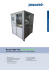### Bio Corey aka Dj Core –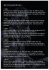### Emergence of spontaneous proto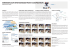### information on the Nutrimill Classic Grain found here!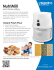### ForAllYouveDone - Happenings At Grace Chapel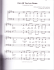### 100 Of the Best Movie Songs Ever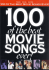### EECS490: Digital Image Processing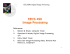### M-900iB_700 - FANUC America